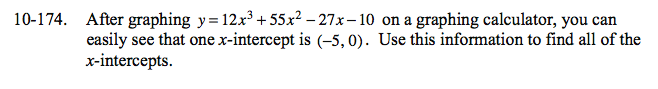Home > CCA2 > Chapter 10 > Lesson 10.3.2 > Problem10-174

10-174.

After graphing y = 12x3 + 55x227x − 10 on a graphing calculator, you can easily see that one x-intercept is (5, 0). Use this information to find all of the x-intercepts. 10-174 HW eTool (Desmos). Homework Help ✎If (−5, 0) is an intercept, then (x + 5) is a factor. Divide the given polynomial by (x + 5), and then use the Quadratic Formula or factor to find the other intercepts.

Use the eTool below to view the graph.
Click the link at right for the full eTool version: CCA2 10-174 HW eTool.